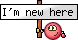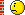Flying Instructors & Examiners A place for instructors to communicate with one another because some of them get a bit tired of the attitude that instructing is the lowest form of aviation, as seems to prevail on some of the other forums!

# mach number and tempratureJoin Date: Dec 2018
Posts: 1
mach number and temprature

hello every one. I have a question. If mach number is achieved at 500 TAS at 10 degrees Celsius, then at 50 degree Celsius how much TAS is required to achieve a mach number. kindly proof answer with the help of mathematical relationship. i will be very thank full to you guys, regardsJoin Date: Feb 2000
Location: UK
Posts: 13,803
The local speed of sound is the square root of gamma * R * T

gamma is the diatomic gas constant, 1.4

R is the gas constant for dry air - 287 kJ/kg.K

T is the temperature in Kelvin.

50 degrees C is 323.15 Kelvin.

SQRT (1.4 x 287 x 323.15) = 360 m/s.

If you prefer knots, divide by 0.5144

And you get c = 700 knots.

If you have a local True Airspeed, divide that by c, and you get the Mach number. So, say you are flying at 500 knots TAS, in an air mass at 50deg.C, then your Mach number is 500/700 = 0.714.

Don't forget that if you are working in IAS or EAS, you need to make further conversions - and in particular at non ISA temperatures, even at sea level, you need to correct the EAS/TAS relationship for density altitude. Ideal gas laws are your friend again here.

So relative density = (pressure / standard sea level pressure) * (standard sea level temperature in K / air temperature in K). And TAS = EAS / SQRT (relative density). Relative density is normally written as sigma.

GJoin Date: May 1999
Location: Quite near 'An aerodrome somewhere in England'
Posts: 25,714
Assuming ISA, +10C static air temp would be at 2525 ft pressure altitude - a TAS of 500KTAS would mean M0.763.

M0.763 at the same pressure altitude at +50C static air temp would imply ISA+40C and would require a TAS of 535KTAS.

Mach No. is the actual TAS divided by the local speed of sound.
Keeping it all simple, TAS = Mach No. x 38.97 x √ absolute static air temp

Last edited by BEagle; 9th Dec 2018 at 11:09.Join Date: Nov 2005
Location: Zulu Time Zone
Posts: 706
As speed of sound is proportional to √absolute temp, it follows that:

The new TAS = 500 x √((50 + 273)/(10 + 273))
= 500 x √(323/283)
= 500 x √1.141
= 500 x 1.07
= 534
Same as BEagle gotJoin Date: Oct 2017
Location: UK
Posts: 1
Why not do it the easy way and use a CRP5 or similar.It took me about 20 seconds to get 535.Join Date: Nov 2005
Location: Zulu Time Zone
Posts: 706
...because the op asked to see the mathsJoin Date: Oct 2007
Location: Here
Posts: 775
If I recall correctly (its been loooong time) the underlying process behind this is quite simple.

Temperature is a measure of the kinetic energy of the motion of the atoms (well, molecules for the gases that comprise air). Kinetic energy is proportional to the square of the velocity of the individual molecules.

E = 1/2 m V*V

Absolute zero is the point at which all motion ceases. This is why temperatures below that cannot exist.

The speed of sound is the result of the average time and distance that a gas molecule travels before bumping into another one. The hotter the gas, the faster the molecules are moving the less time that transpires before a molecule bumps into the next one and the faster the sound pressure wave travels.Join Date: Sep 2003
Location: UK,Twighlight Zone
Posts: 36
Why do we care? Its not a calculation I have done in the cockpit in 10,000hrs. Perhaps we should
concentrate on the job at hand .....Join Date: Feb 2000
Location: UK
Posts: 13,803
Why do we care? Its not a calculation I have done in the cockpit in 10,000hrs. Perhaps we should
concentrate on the job at hand .....
It's certainly a variation on a calculation I've done many times *out of* the cockpit as an aeronautical engineer, and the basic principle of how and why one value varies with another is one that all pilots surely should know - and how do you learn that if not by doing some sample calculations?

GJoin Date: Nov 2005
Location: Zulu Time Zone
Posts: 706
S-Works

Why do we care? It’s not a calculation I have done in the cockpit in 10,000hrs. Perhaps we should
concentrate on the job at hand .....
'We care' because somebody asked the question. That is the job at hand. Also, other people cared enough to figure it out hence you have been able to spend 10,000hrs in a cockpit unbothered by the know-how that made it all possible.

Last edited by oggers; 26th Dec 2018 at 12:22.You may not post new threads
You may not post replies
You may not post attachments
You may not edit your posts

BB code is On
Smilies are On
[IMG] code is On
HTML code is OffShow Printable VersionEmail this Page